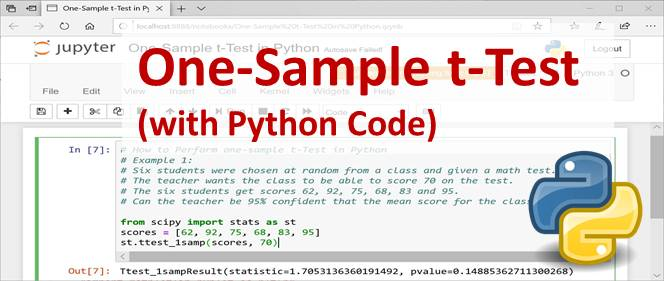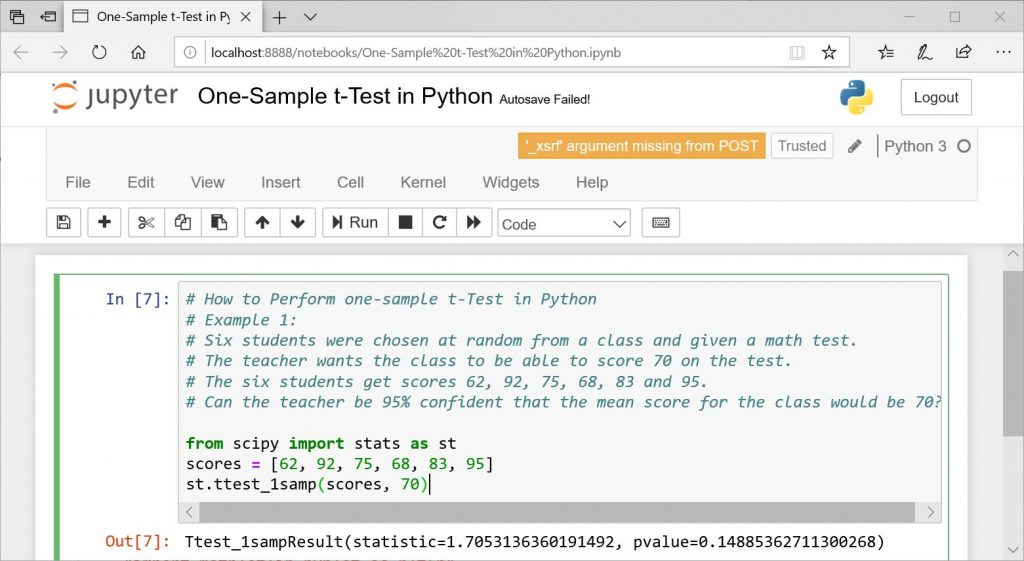# Hypothesis Testing – How to Perform Paired-Sample t-Test (With Python Codes)What is paired-sample t-test?
This is also called two-sample t-test or dependent samples t-test.
This is a test used to compare the means of two populations. Here two samples are provided where corresponding measurements from the two sample could form pairs.This means that the number of measurements from the two samples would be the same.

That is n1 = n2

Scenario where it can be applied
Some of the scenarios where paired t-test could e applied include:
Before-and-after osbservations with the same subject. Examples could be weights of respondents before and and after a weight-loss therapy, test scores of student after taking an intensive prep etc
Another scenario wuold be comparing two different methods of measurement on the same subjects. Example would be comparing the effect of treatment with injection with treatment with tablets on the same group of patients.

How to Carry out Paired-Sample t-Test (Step-by-Step Procedure)
Assuming a sample of n students  were underwent a two-weeks tutorial towards the end of the semester. During this tutorials, past questions and answers were discussed and solved. We want to know how effective the two-weeks tutorial was.
So a test was given to the students before the tutorial and their scores are recorded. After the tutorial, a test was also given to the same set of studend and the scores were recored.
In this case paired sample t-test will help us achieve this objective

Let:
x = test scores of the students before the tutorial
y = scores of the students after taking the tutorial

Step 1: Set up the null and alternate hypothesis

Step 2: Tabulate the given values with columns for difference as shown below
Normally you can use a spreadsheet like excel to to this

Watch a video on how to do this.

Step 3: Calculate the mean difference
To do this, you need to first subtract  the corresponding values for each pair. Then you find the mean of this new column D

Step 4: Calculate the standard deviation of the differences
To to this, you need to subtract the mean difference for each value of D. That would give you the 5th column of the table. Watch the video to get it clearer.
The formula for the standard deviation is:

Remember you need to take square root, to get the standard deviation

Step 5: Calculate the Standard Error
This is given by the formula

Step 5: Calculate the t statistic
The t statistic can be calculated using the formula. That is, the mean difference divided by the standard error value.

Step 6: Look up the t value from table of t-distibution
To to this, you need to know:
the degree of freedom df, given by n-1
where n is the number of samples
Also the significance level, which is normally given. Most times it it 0.05

Step 7: Compare the tabulated t and calculated t
If the calculated value of t is greater than the tabulated value, this means that there is significant difference between the two means. But if the calculated value of t is less than the tabulated value, this means that there is no significant difference between the two.

Your conclusion would be based on the set up of your null and alternate hypothesis. You can either state that: ‘based on the…. we therefore conclude that the tutorials does not have any effect on students performance’ or ‘ we therefore conclude that the tutorials leads to a significant improvement in the performance of students on the test’

Sample Question Solved here

Watch a video on how to use excel to generate mean, Sd and difference.

Python Code for One-Sample t-Test

You can perform paired-samples t-test in Python using the scipy module. The Python Code is shown below:

```# How to Perform one-sample t-Test in Python
# Example 1:
# Six students were chosen at random from a class and given a math test.
# The teacher wants the class to be able to score 70 on the test.
# The six students get scores 62, 92, 75, 68, 83 and 95.
# Can the teacher be 95% confident that the mean score for the class would be 70?

from scipy import stats as st
scores = [62, 92, 75, 68, 83, 95]
st.ttest_1samp(scores, 70)
```

The Output is shown below: